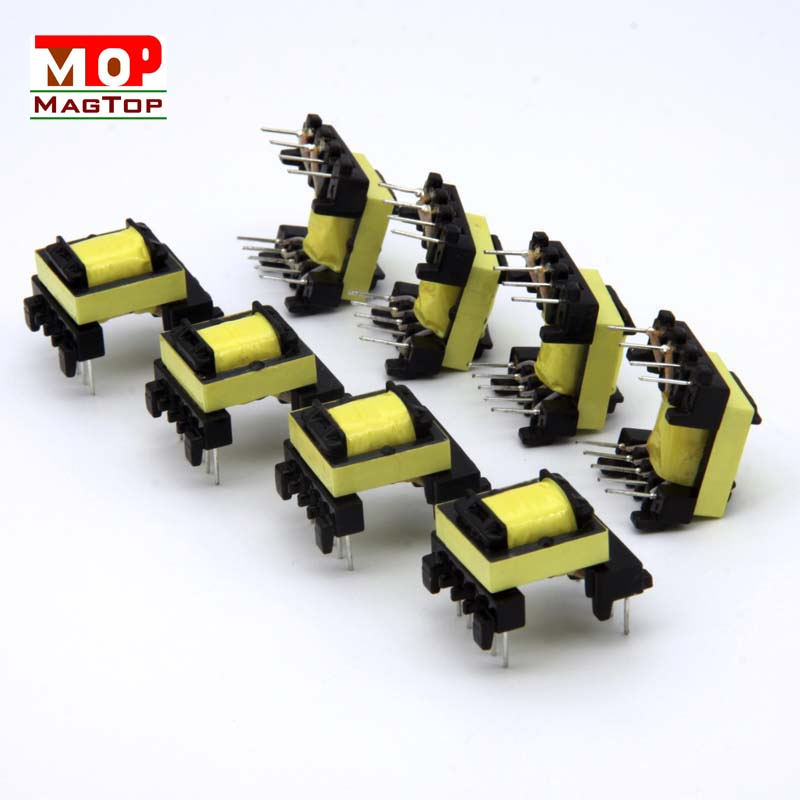# What is the relationship between the number of turns of the transformer coil and the current

##### author: MagTop
01/08/2022In the transformer, the number of turns of the coil determines the magnitude of the current. The direct current is passed into the coil, or the alternating current is passed into the coil. The change of the current size is different. The coil has reactance to the alternating current, and the DC resistance of the coil increases. The larger the current, the smaller the current.

1. The relationship between the number of coil turns and the current

1. Direct current is passed through the coil

According to Ohm's law U=IR, I=U/R, the more coil turns, the greater the DC resistance of the coil and the smaller the current.

2, the coil is fed with alternating current

The coil has reactance to alternating current, and its function is similar to that of resistance. Without considering the direct current resistance, if the frequency is constant, the number of turns of the coil increases, the inductance of the coil will inevitably increase, and the inductive reactance to alternating current will also increase accordingly. The greater the resistance to the AC current, the greater the current will inevitably drop.

The number of coil turns is related to the transformation ratio. Why is it called a transformer? Its main function is to convert the voltage from high voltage to low voltage or from low voltage to high voltage. The ratio of voltage conversion is equal to the ratio of the corresponding coil turns; the current is related to the cross section of the wire , there is a parameter called current density, that is to say, the current capability of a certain wire cross-section of a certain material is the current density. For example, each square millimeter of copper wire can pass 4 amperes of current;

The relationship between the number of transformer turns and the current:

The input to the transformer is proportional to the output voltage and the number of turns.

The total current is also proportional, but not necessarily if the electrical appliance is connected, its general formula is n1i1=n2i2+n3i3.